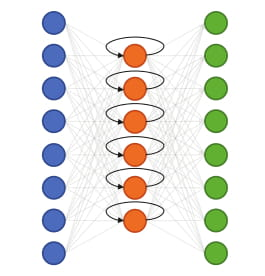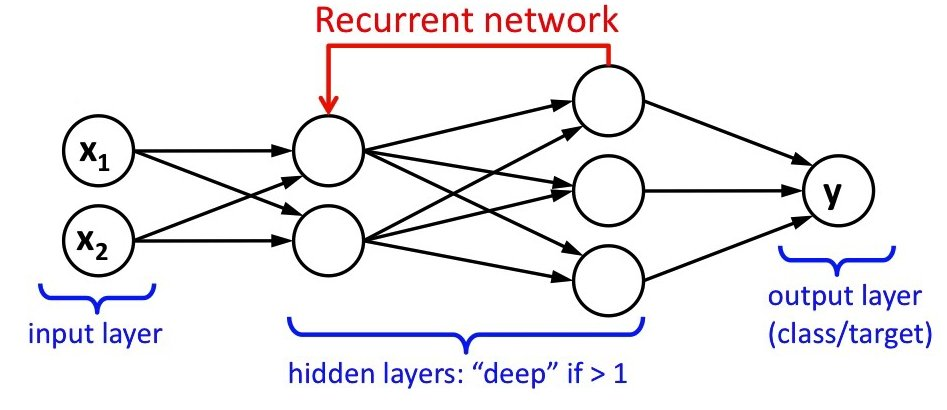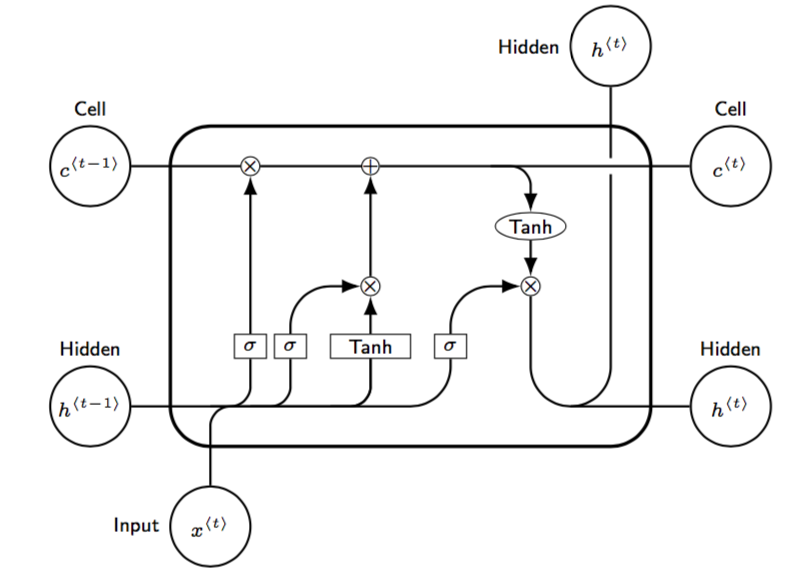×

Search anything:

# Recurrent Neural Networks (RNN)

#### Machine Learning (ML) rnn neural networkGet this book -> Problems on Array: For Interviews and Competitive Programming

Understand Recurrent Neural Networks with an example of assigning an emoji to a sentence

Recurrent Neural Network is one of the widely used algorithms of Deep Learning mainly due to is unique Design. It is the only algorithm that remembers the most recent Input and makes use of memory element. It is used by Apple Siri and Google Voice Search.

Recurrent Neural Network is designed out of a powerful algorithm that uses memory element as part of its training process. RNN was first introduced in the 1980's but can only be shown to there potential since few Years. RNN has internal memory which helps them remember the important feature of most recent input, which helps in the precision in prediction.### Sequential data

Sequential data is a data where related data elements follow each other.

RNN because their memory element can form a much deeper understanding of the sequence of the content given to them they are preferred in:

• time series
• speech
• text
• weather applications

In RNN, the data cycles through a loop. Decision making is based on current input and previous input. For instance, if you provide the network with a word 'hello' while processing 'l' the system would have already forgotten about 'h' so it is not possible to pridict the next word whereas in case of RNN memory element can predict 'o'. Which is different from our previous algorithms.### Long-Short Term Memory

It is an extension for recurrent neural networks, in which the memory is extended. LSTM enables the network to remember inputs information for a longer period of time. A LSTM unit is designed of input gate, output gate and forget gate. The three gates regulate the flow of information over arbitrary interval of time.So to summarize RNN is a robust neural network with the memory element and can provide a great advantage when dealing with sequential data.